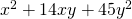Chapter 7: Factoring

# 7.3 Factoring Trinomials where a = 1

Factoring expressions with three terms, or trinomials, is a very important type of factoring to master, since this kind of expression is often a quadratic and occurs often in real life applications. The strategy to master these is to turn the trinomial into the four-term polynomial problem type solved in the previous section. The tool used to do this is central to the Master Product Method. To better understand this, consider the following example.

Example 7.3.1

Factor the trinomial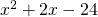.

Start by multiplying the coefficients from the first and the last terms. This is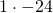, which yields −24.

The next task is to find all possible integers that multiply to −24 and their sums.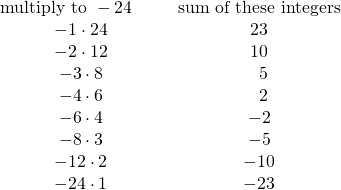Look for the pair of integers that multiplies to −24 and adds to 2, so that it matches the equation that you started with.

For this example, the pair is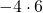, which adds to 2.

Now take the original trinomialand break the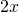into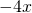and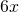.

Rewrite the original trinomial as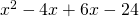.

Now, split this into two binomials as done in the previous section and factor.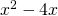yields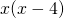and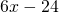yields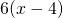.becomes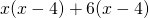.factors to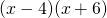.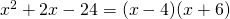Example 7.3.2

Factor the trinomial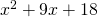.

Start by multiplying the coefficients from the first and the last terms. This is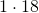, which yields 18.

The next task is to find all possible integers that multiply to 18 and their sums.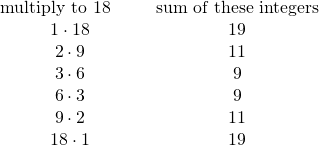Look for the pair of integers that multiplies to 18 and adds to 9, so that it matches the equation that you started with.

For this example, the pair is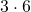, which adds to 9.

Now take the original trinomialand break the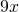into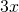and.

Rewrite the original trinomial as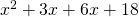.

Now, split this into two binomials as done in the previous section and factor.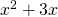yields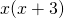and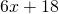yields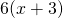.becomes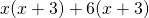.factors to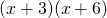.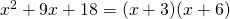Please note the following is also true: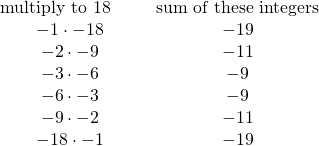This means that solutions can be found where the middle term is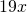,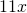,,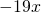,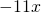or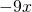.

# Questions

Factor each of the following trinomials.

1.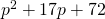2.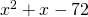3.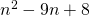4.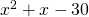5.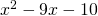6.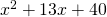7.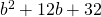8.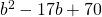9.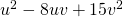10.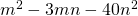11.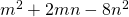12.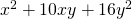13.14.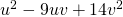15.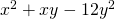16.